# Secant method

(diff) ← Older revision | Latest revision (diff) | Newer revision → (diff)

A method for calculating zeros of continuous functions. Suppose that a zeroof a continuous functionis contained in; let,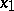be different points of this interval. The iteration formula of the secant method is(1)

If the sequence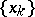converges, then it must converge to a zero of. Ifhas a continuous derivative on, then the local convergence of the secant method to a simple root is super-linear. If the conditions on the smoothness ofare strengthened, one can indicate the exact order of (local) convergence . Namely, for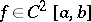andsuch that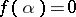,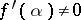,, one has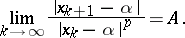Here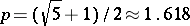, and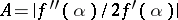.

Super-linear convergence of the secant method for smooth functions is very important since there is no need to calculate the derivatives at each step, only the new value of the function is calculated. Thus, for comparison, in Newton's method (cf. Newton method), whose order of (local) convergence is 2, at each step one has to calculate the values of the function and its derivative; as a rule this is no less laborious than calculating two values of a function.

Since the convergence of the secant method depends on the smoothness of the function and the choice of the initial approximation, in standard computer programs for computing zeros of continuous functions this method is combined with some method for which convergence is guaranteed, for example, the method of bisecting an interval. At each step in such a combined method a rootis located in an interval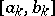at the ends of which the function changes sign (it is assumed that this condition also holds for the initial interval). By a certain test the next approximation is chosen either by (1) or by the formula for bisecting an interval. Ifis smooth, then from some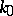onwards the iterations proceed automatically by the secant method. It is possible to have an even more complicated combination of methods, for example, the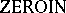algorithm (see ) in which as well as the above-mentioned methods, the method of inverse quadratic interpolation is used. Sometimes by the secant method one means the iteration formula: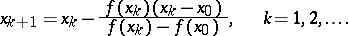(2)

Another terminology for the method (2) is the method of false position, or regula falsi. This method only converges linearly.

In generalizations of the secant method to the case of a system of equations it is possible to consider (1) in two ways. It can be assumed that (1) is obtained from the formula of Newton's method by a discrete approximation of the derivative. Another possibility is to assume that a linear interpolation forat pointsand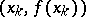has been carried out, and then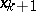is taken to be the zero of the linear interpolant. Both interpretations enable one to obtain a large number of multi-dimensional analogues of the secant method; some of these (but certainly not all) have the same order of (local) convergence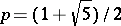(see ).

How to Cite This Entry:
Secant method. Encyclopedia of Mathematics. URL: http://encyclopediaofmath.org/index.php?title=Secant_method&oldid=16449
This article was adapted from an original article by Kh.D. Ikramov (originator), which appeared in Encyclopedia of Mathematics - ISBN 1402006098. See original article